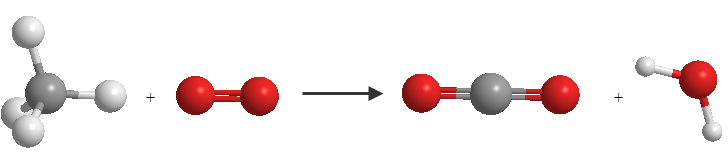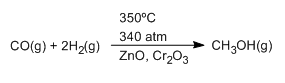In a chemical reaction, a set of substances called reactants are transformed into others called products. A chemical transformation can be evidenced by the change in physical properties of the solution, such as: change in color, release or absorption of heat, formation of precipitates or release of gases.

To describe chemical reactions we use a notation, the chemical equation. The formulas of the reactants are represented on the left side of the chemical equation and those of the products on the right side, both separated by a full-headed arrow in the case of irreversible reactions (which only proceed from left to right) or a double arrow in the case of reversible reactions (they take place in both directions).

$$CH_4+O_2 \rightarrow CO_2 + H_2O$$

This chemical equation represents the combustion reaction of butane. Butane gas (CH 4 ) combines with oxygen (O 2 ) to irreversibly generate carbon dioxide (CO 2 ) and water (H 2 O)In this equation we see that there are different numbers of oxygen and hydrogen atoms on both sides of the equation, which requires an adjustment.

To adjust the hydrogen we put a coefficient 2 in front of the water molecule, so we get the number of hydrogens in reactants and products to be 4.

Next, we adjust for oxygen by placing a coefficient 2 in front of the $O_2$ molecule, thereby equalizing the number of oxygens in reactants and products. The adjusted equation is:

$$CH_4 + 2O_2 \rightarrow CO_2 + 2H_2O$$

The coefficients introduced into the equation during fitting are called stoichiometric coefficients .

In chemical reactions it is usual to indicate the state of aggregation in which reactants and products are found, which are usually: solid (s), liquid (l), gas (g) and aqueous solution (aq). Thus, we can write the combustion of hexane

$$C_{6}H_{14}(l)+\frac{19}{2}O_2(g) \rightarrow 6CO_6(g) + 7H_2O(l)$$

It is also customary to indicate the reaction conditions above or below the reaction arrow. These conditions are temperature, pressure and catalysts used.

The reaction conditions for the methanol synthesis process are indicated below.